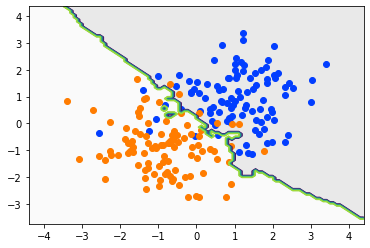In :
import numpy as np
import pylab as plt
import scipy.spatial.distance as scidist

np.random.seed(1234)

In :
import seaborn as sns
sns.set_palette('bright')

In :
def gen_data(N1=100, N2=100):
"""Generates 2D samples from two Gaussians centered at (1, 1) and (-1, -1).

Parameters:
N1 (int): Number of samples from Gaussian centered at (1, 1) labeled as 0
N2 (int): Number of samples from Gaussian centered at (-1, -1) labeled as 1

Returns:
X: Sample data (D, N)
Y: Labels (N,)
"""
X1 = np.random.normal(0, 1, size=(2, N1)) + np.array([, ])
X2 = np.random.normal(0, 1, size=(2, N2)) + np.array([[-1], [-1]])
Y1 = np.zeros((N1,))
Y2 = np.ones((N2,))
X = np.hstack((X1, X2))
Y = np.hstack((Y1, Y2))
print('X contains %d Examples of Class 1 and %d Examples of Class 2 in %d dimensions.'
% (X1.shape, X2.shape, X.shape))
print('Shape of X: %s' % (X.shape, ))
print('Shape of Y: %s' % (Y.shape, ))
return X, Y

In :
print('Generate Train data...')
Xtrain, Ytrain = gen_data()

Generate Train data...
X contains 100 Examples of Class 1 and 100 Examples of Class 2 in 2 dimensions.
Shape of X: (2, 200)
Shape of Y: (200,)

In :
print('Generate Test data...')
Xtest, Ytest = gen_data()

Generate Test data...
X contains 100 Examples of Class 1 and 100 Examples of Class 2 in 2 dimensions.
Shape of X: (2, 200)
Shape of Y: (200,)

In :
def plot_data(X, Y, prefix=''):
for i in range(int(Y.max())+1):
plt.scatter(X[0, Y==i], X[1, Y==i], label='%s Class %d' % (prefix, i))

In :
print('Train data')
plot_data(Xtrain, Ytrain)

Train data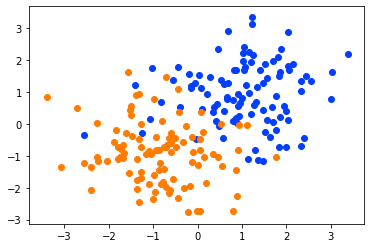In :
print('Test data')
plot_data(Xtest, Ytest)

Test data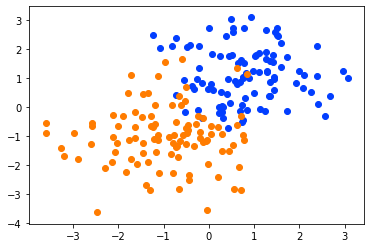In :
"""
Compute distance to all training points, all 3 do the same thing

Parameters:
Xa: shape: (D, N)
Xb: shape: (D, 1)

Returns:
dist: shape: (N,)
"""
def cdist_single_numpy(Xa, Xb):
return np.sqrt(((Xa-Xb)**2).sum(0))

def cdist_single_linalg(Xa, Xb):
return np.linalg.norm(Xa-Xb, axis=0)

def cdist_single_scidist(Xa, Xb):
return scidist.cdist(Xa.T, Xb.T)  # return shape of cdist is (1, 200)

cdist = cdist_single_numpy
# cdist = cdist_single_linalg
# cdist = cdist_single_scidist

In :
def knn_predict_single(Xtrain, Ytrain, Xquery, k=3):
"""Predict the label of a single data point (Xquery) using k-nearest-neighbors.

Returns:
Ypred: Predicted label (1,)
"""
Xquery = Xquery.reshape((Xtrain.shape, 1)) # shape of Xquery is (2, 1), this can broadcast

# compute distance to all training points
dist = cdist(Xtrain, Xquery)

# find k smallest distances (k nearest neighbors)
knn_indices = np.argsort(dist)[:k]

# choose highest voted label
max_vote = np.argmax(ycounts)

return pred_y

In :
# choose a single sample to query
# Xquery = Xtest[:, 0] # shape of Xquery is (2,) this cannot be broadcast together with shape (2, 200)
Xquery = Xtest[:, 0:1] # shape of Xquery is (2, 1), this can broadcast

pred_y = knn_predict_single(Xtrain, Ytrain, Xquery, k=3)

plot_data(Xtrain, Ytrain)
plt.scatter(Xquery, Xquery, s=100, marker='x', label='Query')
plt.legend()
print('Predicted as Class %d' % pred_y)

Predicted as Class 0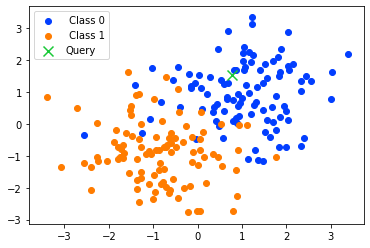In :
def knn_predict_multi(Xtrain, Ytrain, Xquery, k=3):
"""Predict the label of a single data point (Xquery) using k-nearest-neighbors.

Parameters:
Xtrain: Train data, shape: (D, N)
Ytrain: Train labels, shape (D,)
Xquery: Query data, shape: (D, M)

Returns:
Ypred: Predicted label (M,)
"""

# compute distance to all training points, this one works for multiple queries
dist = cdist(Xtrain, Xquery)

# find k smallest distances (k nearest neighbors)
knn_indices = np.argsort(dist, axis=0)[:k, :]

# choose highest voted label
pred_y = (y0count < y1count)

return pred_y

In :
"""
Compute distance to all training points, all 3 do the same thing

Parameters:
Xa: shape: (D, N)
Xb: shape: (D, M)

Returns:
dist: shape: (N, M)
"""
def cdist_multi_numpy(Xa, Xb):
# sqrt(|Xa|^2 + |Xb|^2 - 2 Xa^T Xb)
Xa2 = (Xa**2).sum(0).reshape((-1, 1))
Xb2 = (Xb**2).sum(0).reshape((1, -1))
XaXb = np.dot(Xa.T, Xb)
return np.sqrt(Xa2+Xb2-2*XaXb)

Xa = Xa[:, :, np.newaxis]
Xb = Xb[:, np.newaxis, :]
return np.sqrt(((Xa-Xb)**2).sum(0))

def cdist_multi_scidist(Xa, Xb):
return scidist.cdist(Xa.T, Xb.T)  # return shape of cdist is (M, N)

cdist = cdist_multi_numpy
# cdist = cdist_multi_scidist

In :
pred_y = knn_predict_multi(Xtrain, Ytrain, Xtest, k=3)

plot_data(Xtrain, Ytrain, prefix='Train ')
plot_data(Xtest, pred_y, prefix='Test pred')
plt.legend()

Out:
<matplotlib.legend.Legend at 0x7f2c9f4994a8>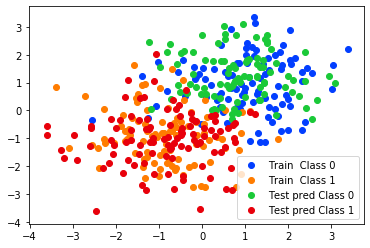In :
def draw_model(Xtrain, Ytrain, k=3):
x = np.linspace(Xtrain.min()-1, Xtrain.max()+1, 100)
y = np.linspace(Xtrain.min()-1, Xtrain.max()+1, 100)
px, py = np.meshgrid(x, y)
p = np.vstack((px.flat, py.flat))

pred_y = knn_predict_multi(Xtrain, Ytrain, p, k)

plt.contourf(x, y, pred_y.reshape(100, 100), int(pred_y.max())+1, alpha=0.1, cmap=plt.cm.gray)
plt.contour(x, y, pred_y.reshape(100, 100), linestyles='-')

In :
k = 1
print('KNN boundary for k = %d' % k)
draw_model(Xtrain, Ytrain, k=k)
plot_data(Xtrain, Ytrain)

KNN boundary for k = 1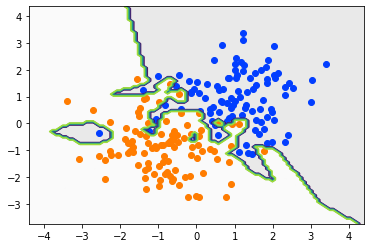In :
k = 3
print('KNN boundary for k = %d' % k)
draw_model(Xtrain, Ytrain, k=k)
plot_data(Xtrain, Ytrain)

KNN boundary for k = 3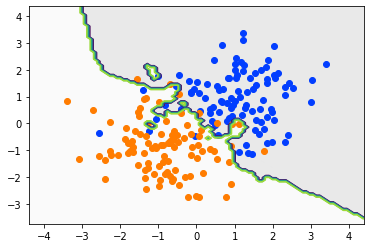In :
k = 5
print('KNN boundary for k = %d' % k)
draw_model(Xtrain, Ytrain, k=k)
plot_data(Xtrain, Ytrain)

KNN boundary for k = 5